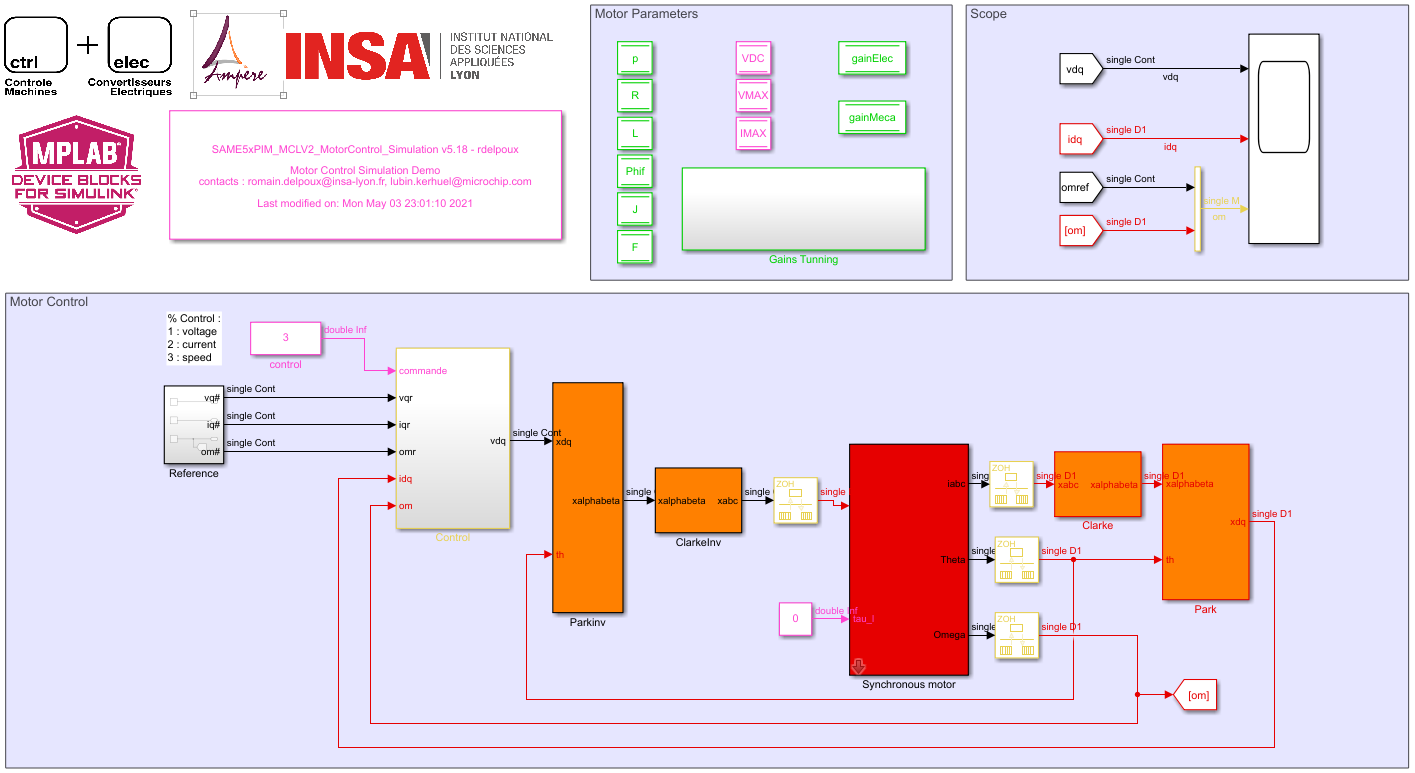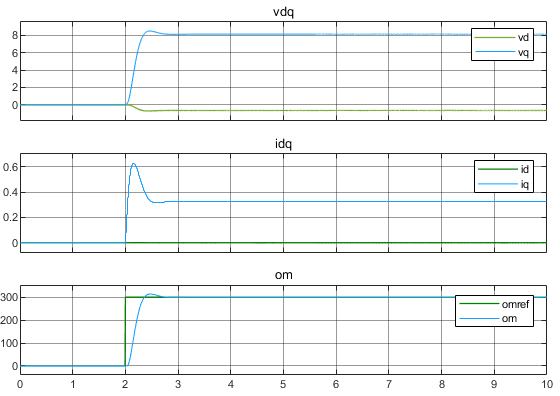# rdelpoux.github.io

Before testing the proposed control on embedded target a simulation of the control strategy apply on a model of the motor is required.

We propose the following simple simulation model :In this simulation file, 3 possible command are proposed.

• Control variable equal to 1, voltage control in the dq reference frame is applied. Applying a vq ref will spin the motor as a DC motor.
• Control variable equal to 2, current control in the dq reference frame is applied. Apply iq ref as an image of the motor torque.
• Control variable equal to 3, speed control in the dq reference frame is applied. In this mode, the simulation result should looks like :